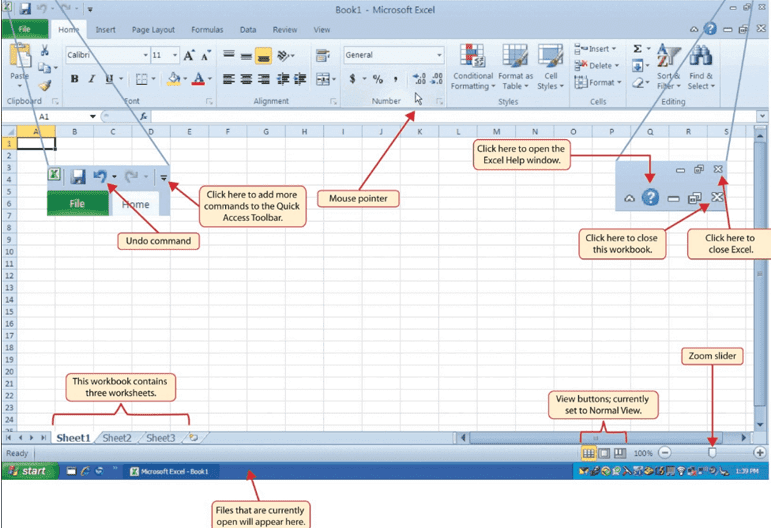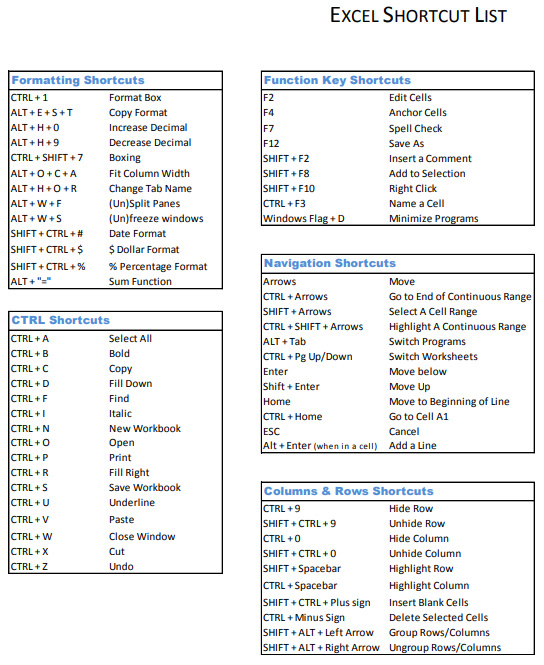# Quick Reference Study Notes for Microsoft Excel << Back to Exam

Microsoft Excel

Microsoft Excel is a spreadsheet computer programme developed by Microsoft for Windows, macOS, Android, and iOS. Microsoft Excel is one among the foremost used software system applications of all time. Hundreds of millions of individuals around the world use Microsoft Excel. You can use excel to enter all types of Data(information) and perform financial, mathematical or statistical calculations.General Uses for Excel
1. Budgets for household or business finances
2. Invoices and receipts
3. Tracking for projects, client and customers, and health records
4. Planners and calendars
6. Financial, loan, debt, and mortgage calculations
7. Inventory management

Basic Excel Terms You Should Know

Workbook and Spreadsheet: A workbook is what you really use once you open excel. The workbook contains the spreadsheets. A book will hold several spreadsheets and you'll be able to move between those sheets with the tabs on the bottom of the excel workbook.

Cell: Spreadsheets are created from rectangular blocks known as cells. A cell contains the data you enter; from numbers to words to pictures to formulas, cells hold that data. You can enter data either directly within the cell or within the formula bar (text box) for the cell right below your ribbon.

Formulas and Functions: You can think about formula as a calculation or equation. With Excel, you'll be able to produce formulas or use inbuilt ones. These formulas will automatically calculate numbers for you wish addition or multiplication.

The Excel Tabs and Ribbon

 Tab Name Description of Commands File Also called the Backstage view of the excel workbook. Contains all commands for opening, closing, saving, and creating new excel workbooks. Includes print commands, document properties, e-mailing options, and help options. The default settings and options also found in this tab. Home Contains the most frequently used Excel commands. Formatting commands are found in this tab with commands for cutting, copying, pasting, and for inserting and deleting rows and columns. Insert Used to insert objects like charts, pictures, shapes, PivotTables, web links, symbols, or text boxes. Page Layout Page layout contains commands that are used to prepare a worksheet for printing. Also includes commands can be used to show and print the gridlines on a worksheet. Formulas Includes commands for adding mathematical functions to a worksheet. Also contains tools for auditing mathematical formulas. Data Used when working with external data sources such as Microsoft® Access®, text files, or the Internet. Also contains sorting commands and access to scenario tools. Review Includes Spelling and Track Changes features. Also contains protection features to password protect worksheets or workbooks. View Used to adjust the visual appearance of a workbook. Common commands include the Zoom and Page Layout view.Excel Formulas

Basic math

 Function Formula Example To add up the total =SUM(Cell range) =SUM(B2+B9) To add individual items =Value1 + Value2 =B2+C2 Subtract =Value1 - Value2 =B2-C2 Multiply =Value1 * Value2 =B2*C2 Divide =Value1 / Value2 =B2/C2 Exponents =Value1^Value2 =B2^C2 Average =AVERAGE(cell range) =AVERAGE(B2:B9) Median =MEDIAN(cell range) =MEDIAN(B2:B9 Max =MAX(cell range) =MAX(B2:B9) Min =MIN(cell range) =MIN(B2:B9)

Simple formatting tricks

 Function Formula Example To change a cell to proper case =PROPER(cell) =PROPER(A2) To change a cell to upper case =Upper(cell) =UPPER(A2) To change a cell to lower case =LOWER(cell) =LOWER(A2)

Conditional statements

 Function Formula Example If statement =IF(logical test, “result if the test answer is true”, “result if the test answer is false”) =IF(B2>54,”Pass,”Fail”) Exact =EXACT(Value1, Value2) =EXACT(B2, C2)

Dealing with dates

 Function Formula Example Return the year =YEAr(datefield) =YEAR(A2) Return the month =MONTH(datefield) =MONTH(A2) Return the day =DAY(datefield) =DAY(A2) Return the day of the week  (1 = Sunday, 2 = Monday, 3 = Tuesday, etc.) =WEEKDAY(datefield) =WEEKDAY(A2) To create a day from a year, month and day =DATE(year, month, day) =DATE(B2, C2, D2)

Pivot Tables
Pivot Tables are used to reorganize information in a spreadsheet. They won't modification the information that you just have, however they will sum up values and compare totally different info in your spreadsheet, depending on what you would like them to do.

To create the Pivot Table, visit information > Pivot Table.

Excel will automatically populate your Pivot Table, however, you can always change around the order of the information.

Then, you have four options to choose from.

Report Filter: this enables you to only look into certain rows in your dataset. For example, if I wanted to create a filter by a house, I could choose to only include students in Gryffindor instead of all students.

Row Labels: These may be your rows within the dataset. Both Row and Column labels can contain information from your columns (e.g. First Name can be dragged to either the Row or Column label -- it simply depends on how you would like to see the information.)

Value: This section permits you to look at your information differently. Instead of simply actuation in any numeric value, you can sum, count, average, max, min, count numbers, or do a few other manipulations with your data. In fact, by default, once you drag a field to value, it always does a count. Since I need to count the number of scholars in every house, I'll go to the Pivot Table and drag the House column to both the Row Labels and the Values. This will add up the number of scholars related to every house.

Row,Column,Cell OperationRow,Column,Cell OperationInsert or delete a column
1. To insert a column, select the column, select Home > Insert >  Insert Sheet Columns.

2. To delete a column, select the column, select Home > Insert > Delete Sheet Columns.

Or, right-click the top of the column, and then select Insert or Delete.

Insert or delete a row
1. To insert a row, select the row, select Home > Insert > Insert Sheet Rows.

2. To delete a row, select the row, select Home > Insert > Delete Sheet Rows.

Or, right-click the selected row, and then select Insert or Delete.

Insert a cell
1. Select one or more cells. Right-click and select Insert.

2. From the Insert box, select a row, column or cell to insert.

Formatting
All cell content uses a similar data formatting by default, which might create it difficult to read a workbook with a lotof data. Basic data formatting can customise the design and feel of your workbook, allowing you to draw attention to specific sections and creating your content easier to look at and understand. You can additionally apply number formatting to inform excel exactly what type of information you’re using within the workbook, like percentages (%), currency (\$), and so on.

In formatting we can:

1. Hide Worksheets

2. Add color to workbook tabs.

4. Add bold, italics, and underlining to text.

6. Change text and cell colors.

7. Change the font and font size.

8. Align text.

9. Wrap text.

10. Indent text.

11. Merge and center text.

12. Change number formats.

13. Change date formats.

14. Show decimals.